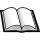Normal view

## Calculus with analytic geometry / John B. Fraleigh.

Publisher: Reading, Mass. : Addison-Wesley, c1980Description: xiii, 865 p. : ill. ; 24 cmISBN: 0201030411 :Subject(s): Calculus | Geometry, AnalyticDDC classification: 515.15,FRA
Contents:
Functions and Graphs (Page-1), The Derivative (Page-27), Differential and Differentials (Page-67), The Trigonometric Functions (Page-97), Applications of the Derivative(Page-113), The Integral (Page-153), Applications (Page-191), Other Elementary Functions (Page-235), Techniques of Integrations (Page-275), Infinite Series of Constants (Page-315), Power Series (Page-351), Plane Curves (Page-397), Polar Coordinates (Page-439), Space Geometry and Vectors (Page-459), Vector Analysis of Curves (Page-509), Differential Calculus of Several variables (Page-537), Applications of Partial Derivatives (Page-573), Multiples Integrals (Page-605), Divetgenous Greens and Stokes Theorems (Page-653), Differential Equations(Page-685), Answers to Odd Number Exercises (Page-753).
Tags from this library: No tags from this library for this title.
Item type Current location Home library Shelving location Call number URL Status Notes Date due Barcode Item holdsBook Military College of Signals (MCS)
Military College of Signals (MCS)
General Stacks 515.15,FRA (Browse shelf) Link to resource Available Almirah No.13, Shelf No.2 MCS7447
Total holds: 0

Functions and Graphs (Page-1), The Derivative (Page-27), Differential and Differentials (Page-67), The Trigonometric Functions (Page-97), Applications of the Derivative(Page-113), The Integral (Page-153), Applications (Page-191), Other Elementary Functions (Page-235), Techniques of Integrations (Page-275), Infinite Series of Constants (Page-315), Power Series (Page-351), Plane Curves (Page-397), Polar Coordinates (Page-439), Space Geometry and Vectors (Page-459), Vector Analysis of Curves (Page-509), Differential Calculus of Several variables (Page-537), Applications of Partial Derivatives (Page-573), Multiples Integrals (Page-605), Divetgenous Greens and Stokes Theorems (Page-653), Differential Equations(Page-685), Answers to Odd Number Exercises (Page-753).

There are no comments on this title.